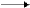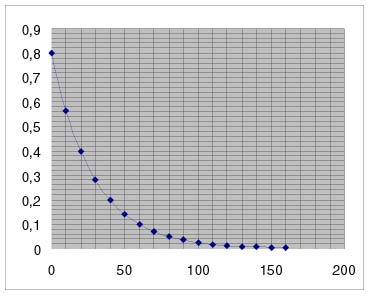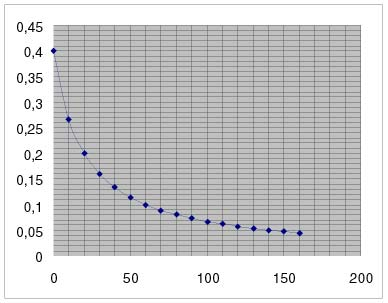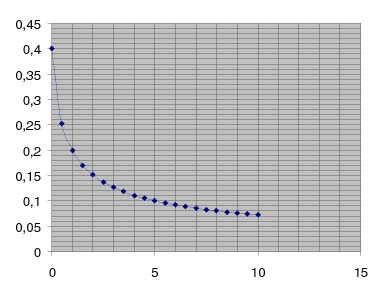MODIFICATION INSTRUCTIONS

In this chapter instructions are given in order to create different arithmetical data and schemes for the exercises, as well as for the creation of new exercises with the desirable prescriptions.
The arithmetical data and schemes should totally agree with the theory types, whether they have been mentioned or not. This is what the scientific ethics impose after all. For this purpose a number of different calculative programs may be used. One of these programs is Excel, by Microsoft, which is installed in most computers. For the use of this program the following specific instructions are given.

EXAMPLE OF HOW TO USE THE PROGRAM EXCEL BY MICROSOFT

First order reactions

In order to find the shape of the curve in first order reactions e.g. AP,  we work out the following familiar type     C = C0e-kt.
In a work sheet of Excel we set at the positions F1 and F2, the prices 0.8 and 0.3465 respectively (we have a satisfactory approach if we give the price 0.0346 at position F2). These prices correspond to the parameters C =0.8 and k=0.03465 of the type C = C0 e-kt .
At the positions from A1 to A17 (more or less may be chosen), we set the prices from 0, 10 up to 160 which correspond to time in seconds. At the position B1 we keyboard the function  =\$F\$1*EXP(-\$F\$2*A1)  which is the insertion of the type  C = 0.8e-0,03465t  in the Excel program, then we press "enter". We repeat the procedure at the positions B2 to B17. The program has the capability of automatic repetition. The columns A and B result as shown in the following table. The next step is to give directives in order to design the diagram.

 A B C D E F G H I G 1 0 0.8 0.8 2 10 0.565727 0.03465 3 20 0.4000594 30 0.282905 5 40 0.200059 6 50 0.141473 7 60 0.100044 8 70 0.070747 9 80 0.050029 10 90 0.035379 11 100 0.025018 12 110 0.017692 13 120 0.012511 14 130 0.008847 15 140 0.006256 16 150 0.004424 17 160 0.003129

The curve that arises corresponds to a first order reaction, this is obvious from the time that the it takes for the concentration to decrease to one-half its initial value. Every 2 seconds, C decreases one-half its initial value, as the following type predicts,    t1/2 = 0.693 / k     for first order reactions.
By changing the prices at the positions F1 and F2 we create curves with desirable prescriptions (in the Excel program).
If we want to create the above curve only, we keyboard at the position B1 the function with the simplified form:  =0.8*EXP(-0.03465*A1)   and continue in a similar way.
For the creation of nicer schemes with even more choices, we can use another program, Illustrator by Adobe, having as a guide the curve that we created in Excel, since Illustrator is not a calculative program. In order to achieve this, we transfer Excel’s scheme in Illustrator using the directions copy and paste, we work out the scheme in order to isolate the curve, which then we transfer to the appropriate lattice axis. We can also give prices that we took from Excel program. As an example we refer the scheme of exercise 10 as well as in the chapter “Data of simple reactions with diagrams”.

Second order reactions

For the shape of a second order reaction curve e.g. 2AP, we work out the familiar type C = C0 / (1+ktC0).
In a n Excel’s work sheet we set at the positions F1 and F2, the prices 0.4 and 0.125 respectively. These prices correspond to the parameters C0 = 0.4 and k = 0.125 of the type C = C0 / (1+ktC0). We remind that independently of the number of the reactants the shape of the curve is the same.
At the positions from A1 to A18 (more or less positions can be chosen), we set the prices from 0 to 17 which correspond to time in seconds. At the position B1 we keyboard the function  =\$F\$1/(1+\$F\$2*A1*\$F\$1)   which is the insertion of the type  C =  0.4 / (1+0.125t0.4) in the Excel program, then we press "enter". We repeat the procedure at the positions B2 to B17. Thus, the columns A and B result in the following table. Afterwards we give directives in order to design the diagram.

 A B C D E F G H I G 1 0 0.4 0.4 2 10 0.266667 0.125 3 20 0.24 30 0.16 5 40 0.133333 6 50 0.114286 7 60 0.1 8 70 0.088889 9 80 0.08 10 90 0.072727 11 100 0.066667 12 110 0.061538 13 120 0.057143 14 130 0.053333 15 140 0.05 16 150 0.047059 17 160 0.044444

The resulting curve seems to correspond in a second order reaction, as we can see from the time it takes to decrease to one half its initial value. This first decrease takes place after 20 seconds. The second decrease, once again to one half its previous value, takes place 40 seconds later from the first, which is 20+40=60 seconds since initiation and the fourth takes place 140 seconds since initiation. These prices are predicted from the half life time which has the type
t1/2 = 1 / kCfor second order reactions. 1 /0.125.0.4 = 20
1 / 0.125.0.2 = 40.
For the creation of schemes in a specialized schemes program we follow the procedure that was mentioned in the case above (first order reactions). As an example we refer to the  solution scheme of exercise 21. as well as in the chapter “Data of simple reactions with diagrams”.

Third order reactions

In order to predict the shape of the curve of a third order reaction e.g. 3AP,
we work out the familiar type C = C0 / (1+2ktC02)0.5
In a n Excel’s work sheet we set in the positions F1 and F2, the prices 0.4 and 9.375 respectively. These prices correspond to the parameters C0 = 0.4 and k = 9.375 of the type C = C0 / (1+2ktC02)0.5
We remind that independently of the number of the reactants the shape of the curve is the same.

At the positions A1 to A19, we set the prices from 0 to 19 which correspond to time in seconds. At the position B1 we keyboard the function

=\$F\$1/(1+2*\$F\$2*A1*\$F\$1^2)^0.5, which is the insertion of the type
C =  0.4 / (1+2
.9.375t0.42)0.5  in Excel program and then we press "enter". We repeat the procedure at the positions B2 to B19. Therefore, the columns A and B result in the following table. Finally we give directives in order to design the diagram.

 A B C D E F G H I J 1 0 0.4 0.4 2 0.5 0.252982 9.375 3 1 0.24 1.5 0.170561 5 2 0.151186 6 2.5 0.137199 7 3 0.126491 8 3.5 0.117954 9 4 0.11094 10 4.5 0.105045 11 5 0.1 12 5.5 0.095618 13 6 0.091766 14 6.5 0.088345 15 7 0.08528 16 7.5 0.082514 17 8 0.08 18 8.5 0.077703 19 9 0.075593

Once again from the time that it takes for the concentration to decrease to one half its initial value we can ascertain that the curve corresponds in a third order reaction. The first decrease of the concentration to one half its initial value takes place 1 minute later and the second 4 minutes later from the first, which is 1+4=5 minutes since initiation. These prices are predicted from the half life time which has the type

t1/2 = 1,5 / kC02    for third order reactions 1.5 /9.375.0.42 = 1
1.5 / 9.375.0.22 = 4

As an example we refer to the chapter “Data of simple reactions with diagrams”.